# 1. Below are production functions that turn capital (K) and labor (L) into output. For each...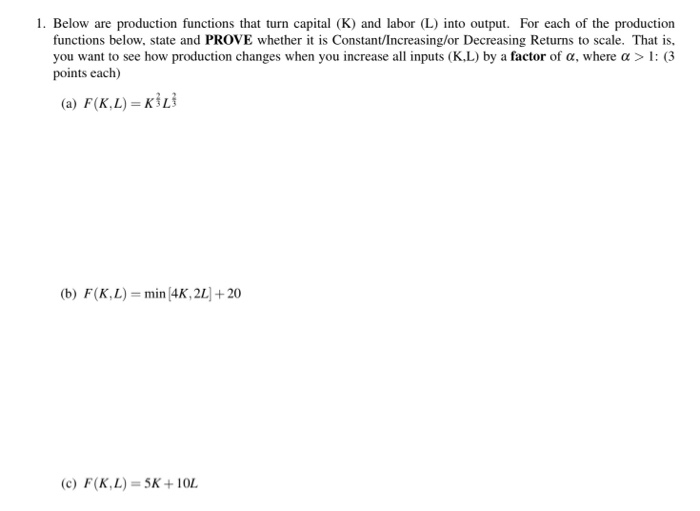1. Below are production functions that turn capital (K) and labor (L) into output. For each of the production functions below, state and PROVE whether it is Constant/Increasing/or Decreasing Returns to scale. That is, you want to see how production changes when you increase all inputs (KL) by a factor of a, where a > 1: (3 points each) (a) F(K.L) = (b) F(KL)= min (4K, 2L + 20 (c) F(K,L) = 5K+ 10L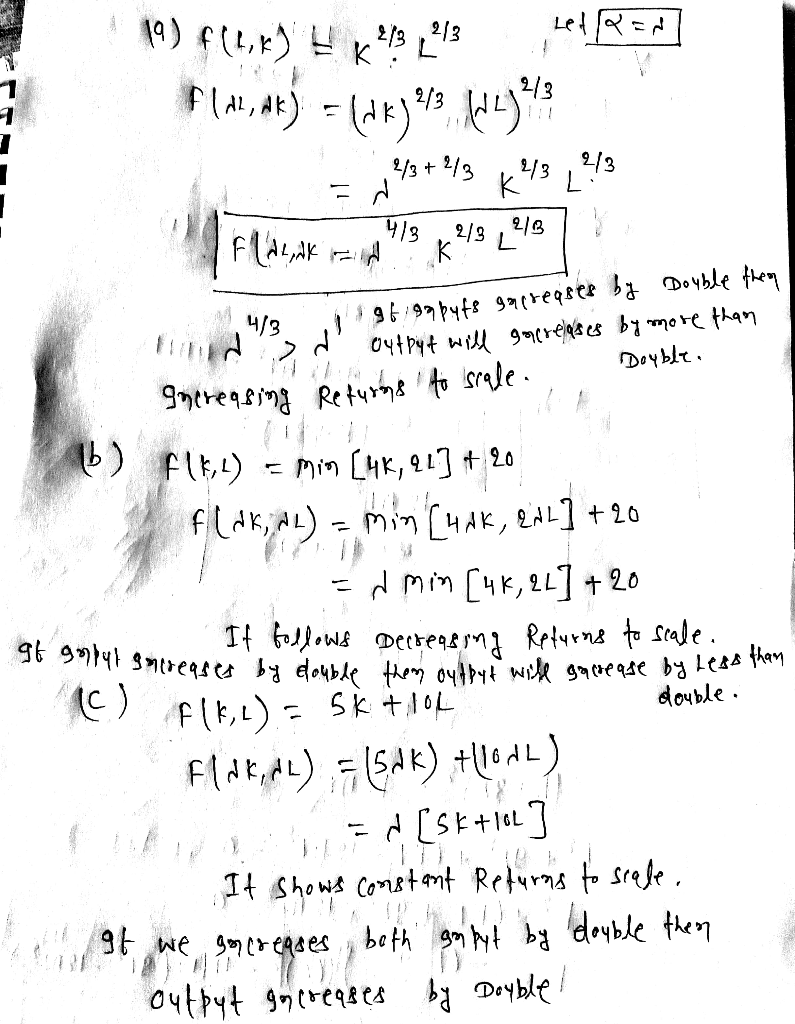#### Earn Coin

Coins can be redeemed for fabulous gifts.

Similar Homework Help Questions
• ### 1. Below are production functions that turn capital (K) and labor (L) into output. For each...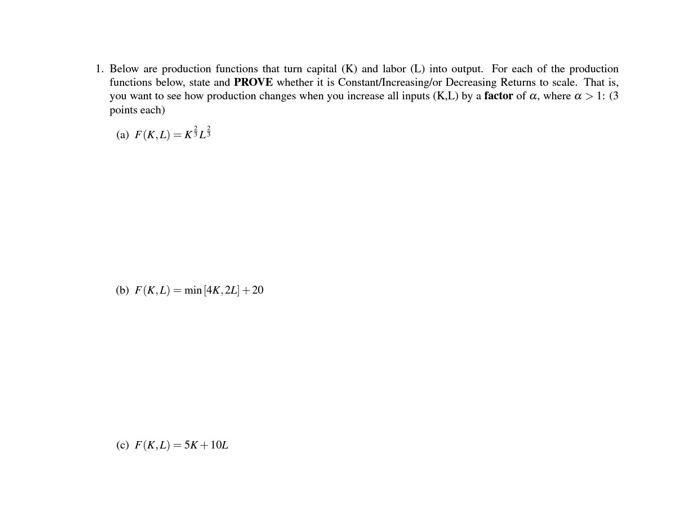1. Below are production functions that turn capital (K) and labor (L) into output. For each of the production functions below, state and PROVE whether it is Constant/Increasing/or Decreasing Returns to scale. That is, you want to see how production changes when you increase all inputs (K.L) by a factor of a, where a > 1: (3 points each) (a) F(KL)=KL (b) F(K,L) = min (4K, 22] + 20 (c) F(K,L) = 5K+10L

• ### 4. Below are production functions that turn capital (K) and labor (L) into output. For each...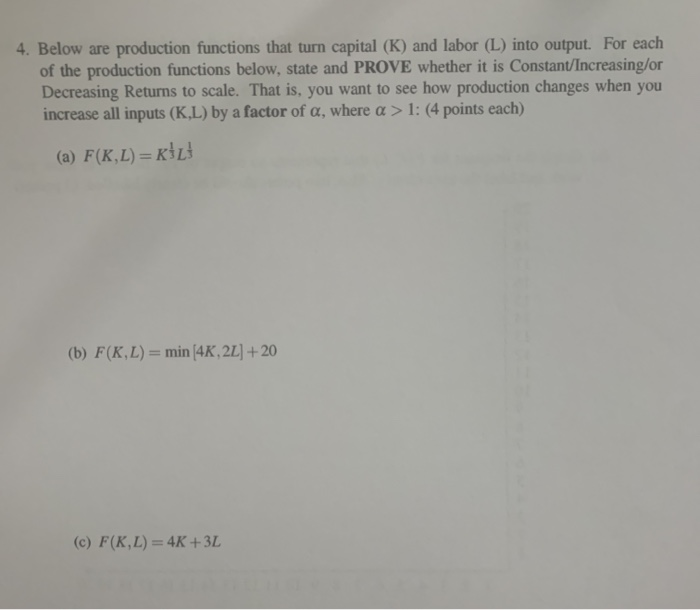4. Below are production functions that turn capital (K) and labor (L) into output. For each of the production functions below, state and PROVE whether it is Constant/Increasing/or Decreasing Returns to scale. That is, you want to see how production changes when you increase all inputs (K.L) by a factor of a, where a > 1: (4 points each) (a) F(K,L) =KİL (b) F(K,L) = min 4K, 2L] + 20 (c) F(K,L) = 4K +3L 5. For this problem you...

• ### 1. Below are production functions that turn capital (K) and labor (L) into output. For cach of th...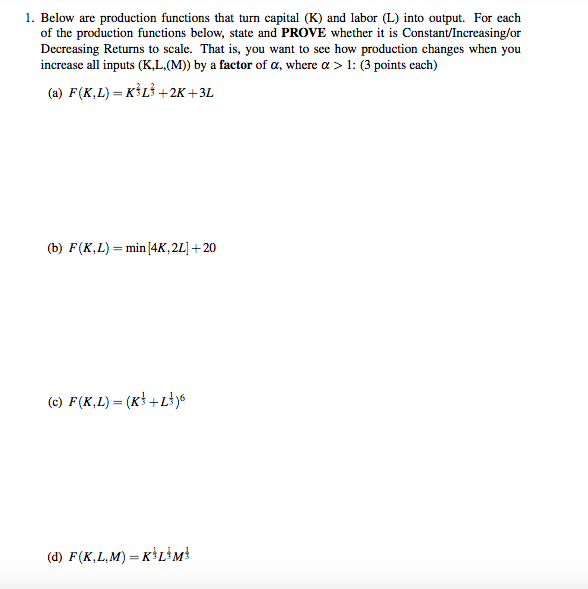1. Below are production functions that turn capital (K) and labor (L) into output. For cach of the production functions below, state and PROVE whether it is Constant/Increasing/or Decreasing Returns to scale. That is, you want to see how production changes when you increase all inputs (K,L, (M)) by a factor of a, where a > 1: (3 points each) (a) F(K,L)-KİLİ+2K +3L (b) F(K, L)=min/4K, 2L1+20 (d) F(K,L,M) KL3M 1. Below are production functions that turn capital (K) and...

• ### Below are production functions that turn capital (K) and labor (L) into output. For each of...

Below are production functions that turn capital (K) and labor (L) into output. For each of the production functions below, state and PROVE whether it is Constant/Increasing/or Decreasing Returns to scale. That is, you want to see how production changes when you increase all inputs (K,L,(M)) by a factor of α, where α > 1: (4 points each) a) F(K,L) = K^1/3*L^1/3+2K+3 b) F(K,L) = sqr(K^3+L^3) c) F(K,L) = (K^2/4+L^2/4)^2 d) F(K,L,M) = min(K,L)*M

• ### Determine whether each of the production functions below displays constant, increasing, or decreasing returns to scale:...

Determine whether each of the production functions below displays constant, increasing, or decreasing returns to scale: Q = K + L + KL Q = 2K2 + 3L2 Q = KL Q = min(3K, 2L)

• ### Determine whether each of the production functions below displays constant, increasing, or decreasing returns to scale:...

Determine whether each of the production functions below displays constant, increasing, or decreasing returns to scale: Q = 10K0.75L0.25 Q = (K0.75L0.25)2 Q = K 0.75L0.75 Q = K 0.25L0.25 Q = K + L + KL Q = 2K2 + 3L2 Q = KL Q = min(3K, 2L)

• ### 4. Proving constant returns to scale A production function expresses the relationship between inputs, such as...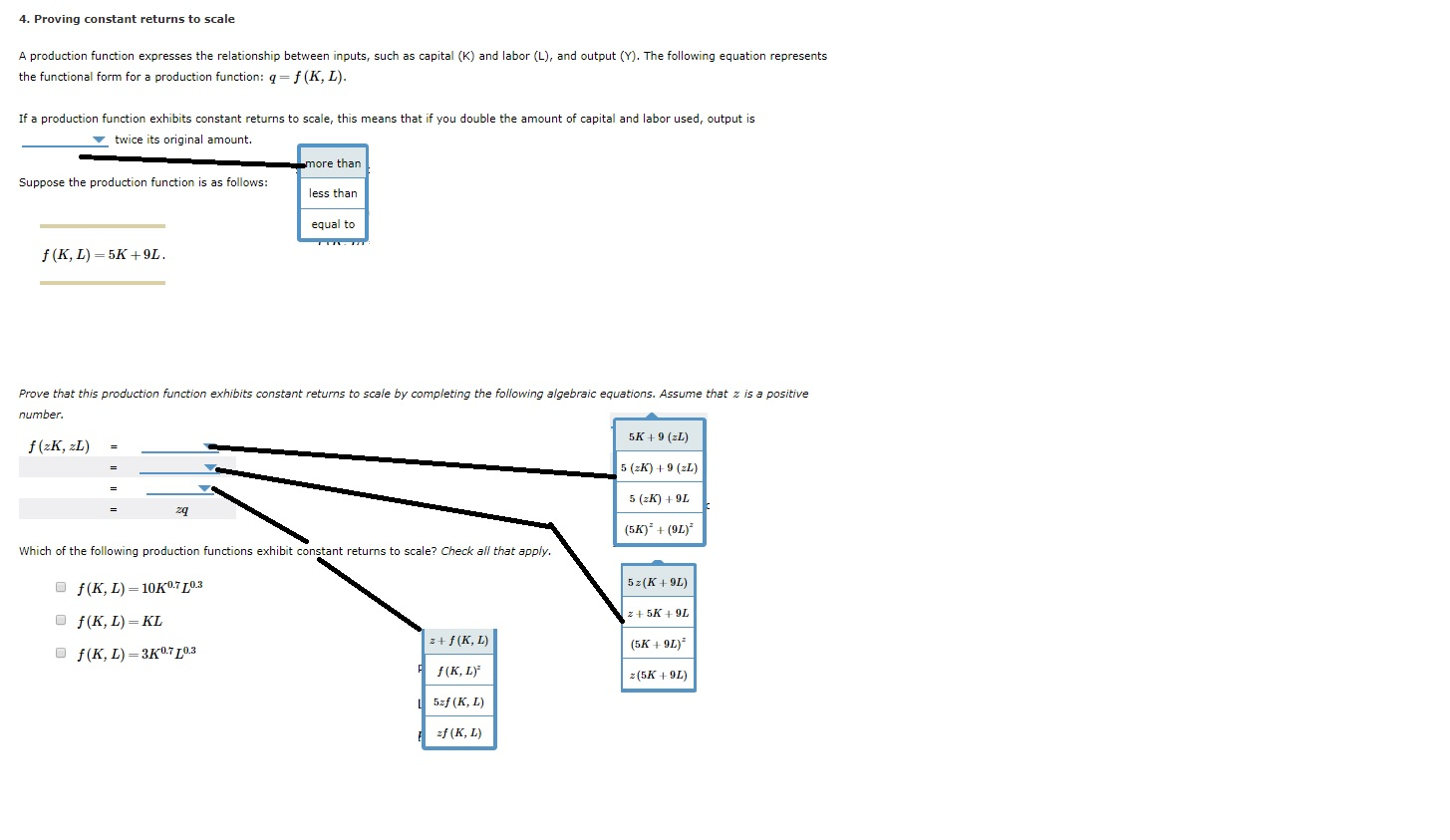4. Proving constant returns to scale A production function expresses the relationship between inputs, such as capital (K) and labor (L), and output (Y). The following equation represents the functional form for a production function: 9=f(K, L). If a production function exhibits constant returns to scale, this means that if you double the amount of capital and labor used, output is twice its original amount. more than Suppose the production function is as follows: less than equal to f( KL)=5K+9L...

• ### 3. Determine whether each of the following production functions below displays constant, increasing, or decreasing returns...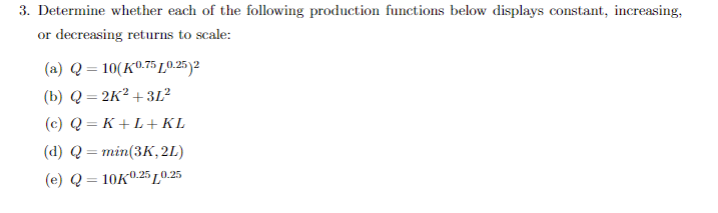3. Determine whether each of the following production functions below displays constant, increasing, or decreasing returns to scale: (a) Q = 10(K0.75 0.252 (b) Q = 2K2 +312 (c) Q=K+L+KL (d) Q = min(3K, 2L) (e) Q = 10K0:250.25

• ### 1. A production function is given by f(K, L) = L/2+ v K. Given this form,...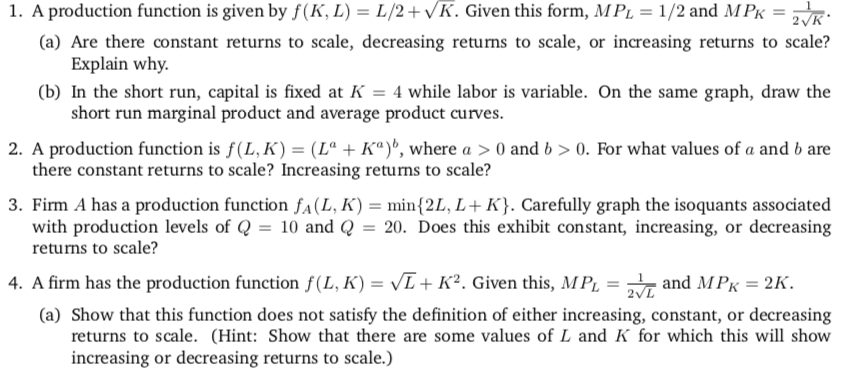1. A production function is given by f(K, L) = L/2+ v K. Given this form, MPL = 1/2 and MPK-2 K (a) Are there constant returns to scale, decreasing returns to scale, or increasing returns to scale? (b) In the short run, capital is fixed at -4 while labor is variable. On the same graph, draw the 2. A production function is f(LK)-(L" + Ka)", where a > 0 and b > 0, For what values of a and...

• ### 1. Graph the short-run total product curves for each of the following production functions if K...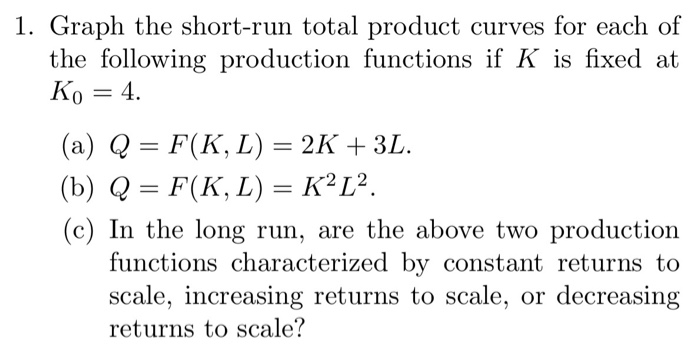1. Graph the short-run total product curves for each of the following production functions if K is fixed at Ko 4 (a) Q = F(K, L) = 2K + 3L. (b) Q = F(K, L) = K2L2. (c) In the long run, are the above two production functions characterized by constant returns to scale, increasing returns to scale, or decreasing returns to scale?

Free Homework App